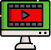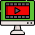VideosNotes

### Quadratic Equations. (A) - 1

Quadratic Equations.
A quadratic equation is an equation of the second degree, meaning it contains at least one term that is squared. The standard form is ax² + bx + c = 0 with a, b, and c being constants, or numerical coefficients, and x is an unknown variable.
Views: 3
Video Duration: 11:56 (Part 1 of 4)
Date Uploaded: November 05, 2020
Grade Level: SS 2
Share this with your friends!
Continue the Series...On the Same TopicQuadratic Equation
A quadratic equation is an ...
Other Related Videos